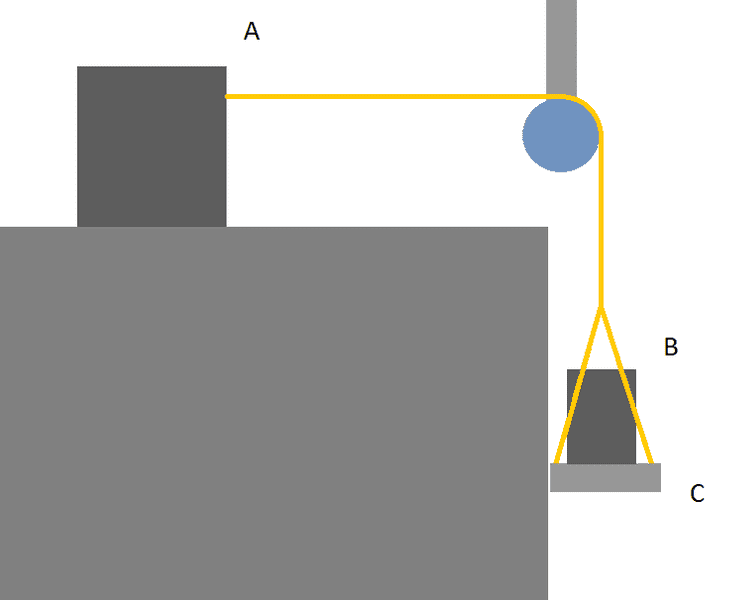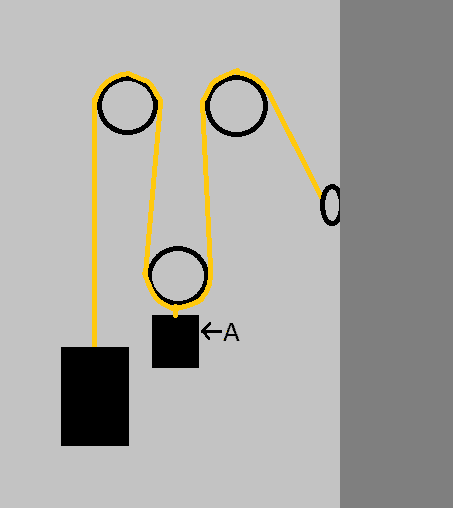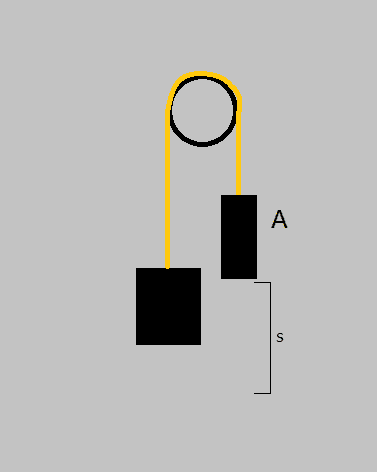# Acceleration of a system due to gravity

The problem was present in a physics 1 exam, and I'm reasonably sure I know the answer, yet my friend contradicts me in my conclusion.

## Homework Statement

Find magnitude of acceleration of system ABC. Masses of A, B and C are all equal and each has mass 2.00 kg. Let gravitational acceleration g = 9.81 m/s2. See figure below.Disregard friction - castor, air, and surface.

## Homework Equations

Newtons 2nd law of motion:
F = m a (1)

## The Attempt at a Solution

The force on the whole system is gravity's effect on B and C (weight of B and platform C). Through (1):

F = (2)(2.00 kg)(9.81 m/s2) = 39.24 N

Acceleration on the whole system is then, under the above force: (Through (1) again):

a = (39.24 N)/[(3)(2.00 kg)] = 6.54 m/s2.

Obviously, the acceleration of A is directed horizontally, while B and C are accelerating downwards. Still, the acceleration of each objects will be the same, yet in their respective directions. It is this value which I am after.

Also, can someone less ignorant than I tell me if I am right in this:
Without other external forces (other than gravity), the system will never accelerate faster than 9.81 m/s2 and the less the mass of A (and the larger the mass of B and C, equivalently), the closer the systems acceleration will be to 9.81 m/s2.

Thank you in advance and I apologize for the triviality of the problem :s

Bystander
Homework Helper
Gold Member
Your analysis is complete and correct for the information you're wanting about the system. Any other questions?

Doc Al
Mentor

Thanks for the (very) quick replies!

Your analysis is complete and correct for the information you're wanting about the system. Any other questions?

If you don't mind, continuing on the theme of triviality; Could I make something accelerate faster than gravity using wheels and cables, like so (A):Again, disregarding frictions. How should I think when finding acceleration and tensions in these kinds of "problems?"

Bystander
Homework Helper
Gold Member
If "A" is the larger mass, the unlabeled mass can be accelerated at greater than g; you've got the double (one and a halfle?) advantage with the pulley set-up. Think of an asymmetric see-saw, or catapult, with a large mass on the short end and a small mass on the long. Trebuchet. Might be a variety of other gravity driven "siege engines."

Okay, so comparing my previous image to the one below:Here, gravity's pull on A (again, like you did, assuming that A's mass is greater than that of the unlabeled), displacing A the distance s, will do the same to the unlabeled weight. In the previous image, displacing A by s, would displace the weight by 2 times s (?). I assume it is harder to displace A in the previous image, then, seeing as the work done by gravity is double there?

Bystander